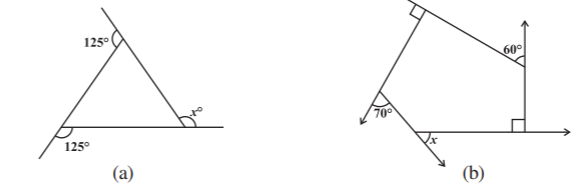Open in App
Not now

# Class 8 NCERT Solutions – Chapter 3 Understanding Quadrilaterals – Exercise 3.2

• Last Updated : 15 Dec, 2020

### Question 1. Find x in the following figures.#### Solution:

As we know , the sum of the measures of the external angles of any polygon is 360°.

(a) 125° + 125° + x = 360° ⇒ 250° + x = 360° ⇒  x = 110°

(a) 70° + 60° + x + 90° + 90° = 360° ⇒ 310° + x = 360° ⇒  x = 50°

### (i) 9 sides

#### Solution:

Measure of angles = 360°/ 9 = 40°

As in a regular polygon all interior angle are equal so all the exterior angles will also be equal.

### (ii) 15 sides

#### Solution:

Measure of angles = 360°/ 15 = 24°

### Question 3. How many sides does a regular polygon have if the measure of an exterior angle is 24°?

#### Solution:

Given measure of exterior angle = 24°

(no. of sides) x (measure of exterior angle) = 360° ⇒ no. of sides = 360°/24° = 15

### Question 4. How many sides does a regular polygon have if each of its interior angles is 165°?

#### Solution:

Given measure of interior angle = 165°

measure of exterior angle = 180° – 165° = 15°

(no. of sides) x (measure of exterior angle) = 360° ⇒ no. of sides = 360°/15° = 24

### (b) Can it be an interior angle of a regular polygon? Why?

#### Solution:

(a) given here, measure of exterior angle = 22°

As we know, (no. of sides) x (measure of exterior angle) = 360°

putting here value, we get

No. of sides = 360°/22° ≈ 16.36 (approx), which is never possible .

Number of sides can never be fractional.

(b) No. It cannot be an interior angle of a regular polygon.

In this case , measure of exterior angle will be = 158°

again we will get fractional no. of sides. Hence not possible.

### (b) What is the maximum exterior angle possible for a regular polygon?

#### Solution:

(a) An Equilateral Triangle is a regular polygon with minimum number of sides because all sides are equal in it. We know that each angle of an equilateral triangle measures 60° . Hence, 60° is the minimum possible value for internal angle of a regular polygon.

(b) Each exterior angle of an equilateral triangle is 120° and hence this the maximum possible value of exterior angle of a regular polygon. This can also be proved by another principle; which states that each exterior angle of a regular polygon is equal to 360° divided by number of sides in the polygon. If 360° is divided by 3, we get 120° .

My Personal Notes arrow_drop_up